# Analytics Calculations: Date Functions

Moderator, Lightspeed Staff Posts: 66 moderator
edited May 2019
*Note, from this part of the Discussions, we will assume that you're now comfortable with the points of Calculations. Changing dimensions and measures on reports, changing filters on reports, finding the Calculations button, finding dimensions and measures in calculations, and some of the basic starter calculations: addition, subtraction, division, multiplication, greater than, less than, equal to, single-criteria IF functions, and multiple criteria IF functions.

If you're in the early stages of your Analytics mastery, you may want to take a quick look at the related Discussions:

Multiplying by percentages in Analytics

Analytics provides lots of functions that perform different mathematical, logical, and table-based calculations. You can find a more in-depth catalogue of available functions on the Looker list of functions and operators.

To make it easier, we could categorize these in five different ways: Cell functions, Date functions, Logic functions, Math functions, and Table functions.

In this article, we'll be looking at seven of the Date based functions:
Add_Days, Date, Diff_Days, Extract Day, Now, To_Date, Trunc_Days

# Add_Days (or Weeks, Months, Years)

Looks at a date field and then changes it by a defined number (one day is equivalent to the number 1)

The first argument is the number difference. This can be positive or negative.
The second argument is the date that you wish to adjust.

In this example, I am creating two calculations, one to change the sales day forward by one day, the second to change the sales day backwards by one day: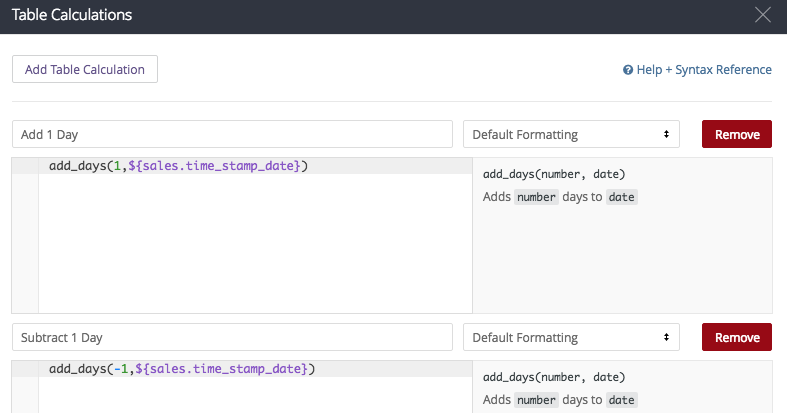and if I've built them properly: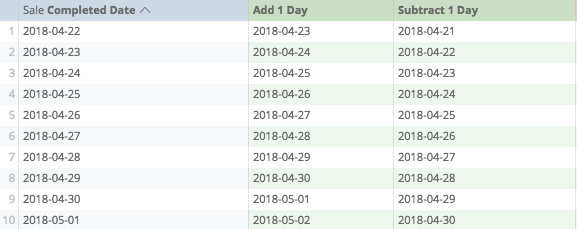# Date

Creates a date out of provided numbers.

Requires three arguments,

• The first argument is the number for the year.
• The second argument is the number of the month (the number one corresponding to January, the number two corresponding to February and so on)
• The third argument is the number of the day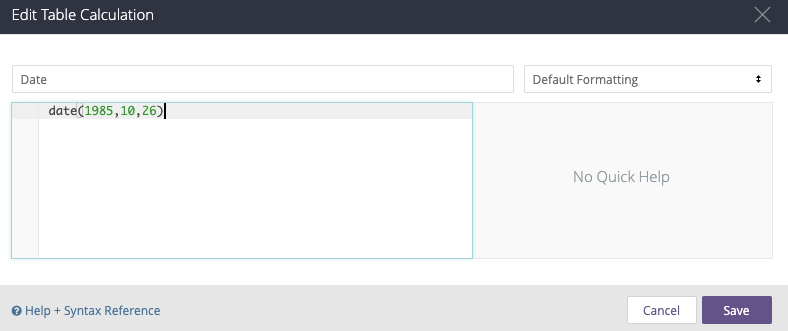Becomes illustrated as# Diff_Days (or Weeks, Months, Years)

Counts the difference in days (or other denominations) between two dates.

To prepare it, the first argument is the start date (or earlier date), the second is the end date (or later date)

*Note, this logic is different from how spreadsheets may calculate differences between dates, where you might subtract a smaller from a larger to yield a positive number.

If I wanted to count the difference from the start of the month to the current sales date, I would prepare my calculation to look like: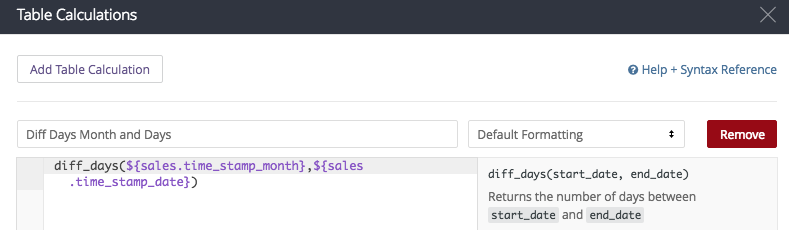My calculation is:
diff_days(\${sales.time_stamp_month},\${sales.time_stamp_date})

And if I have done my calculation properly: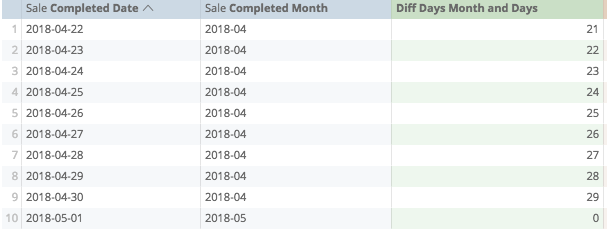# Extract_days (or Months, Years)

Converts part of a date to a non-date-number:

Example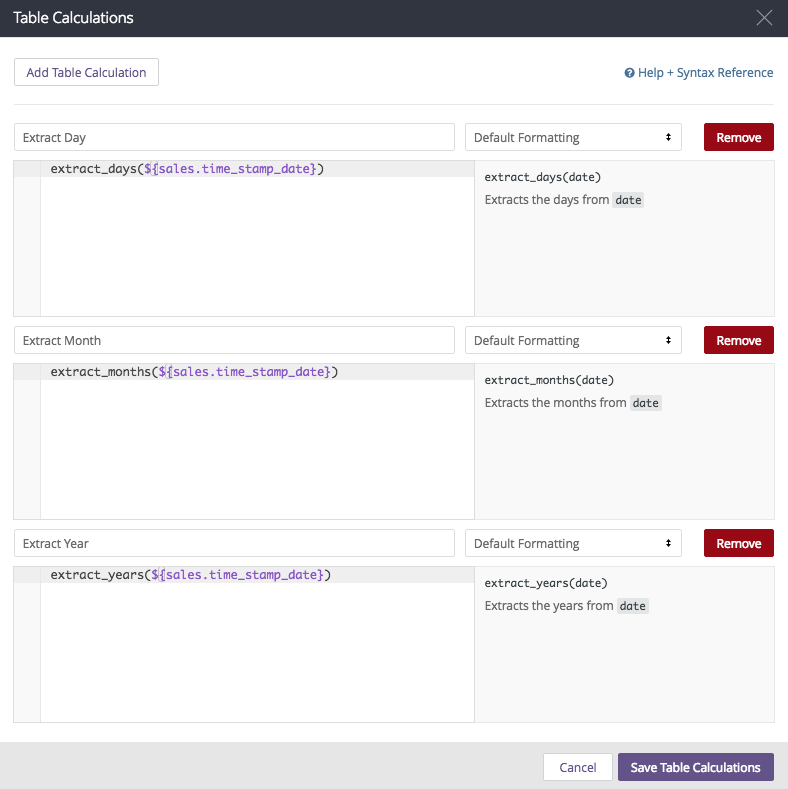My calculations look like:

extract_days(\${sales.time_stamp_date})
extract_months(\${sales.time_stamp_date})
extract_years(\${sales.time_stamp_date})

and if I have prepared my calculations properly...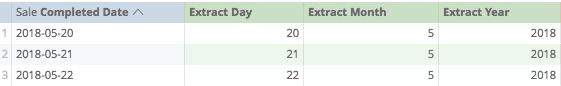# Now

Returns current timestamp, no other arguments required.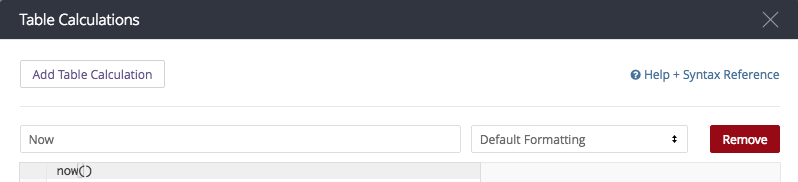In this instance, it looks like:
now()

and if I have saved it properly...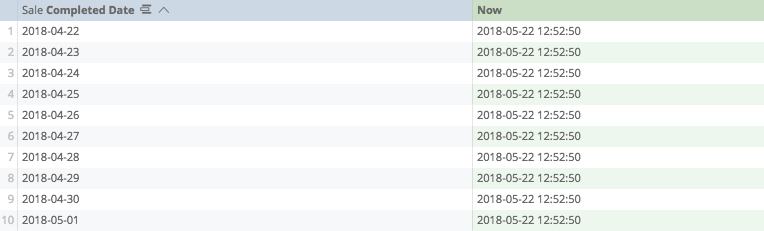# To_date

Changes a string of text-numbers to a date, and must follow one of the following formats:

YYYY
YYYY-MM
YYYY-MM-DD
YYYY-MM-DD hh
YYYY-MM-DD hh:mm
YYYY-MM-DD hh:mm:ss

*Note: The year 2018, the number 2,018 and the word 2018 are not considered the same by Analytics. In this instance, it is the word 2018 that responds to the to_date function.

In this calculation, I recreated the date from straight text: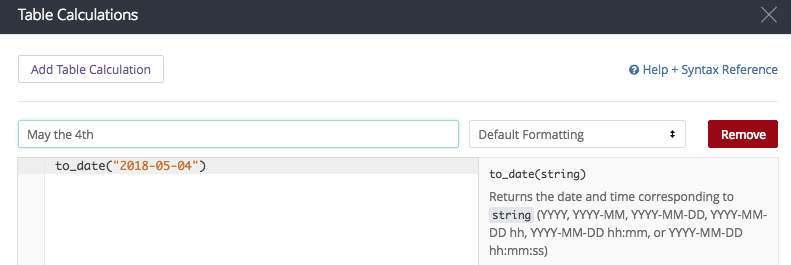The calculation being
to_date("2018-05-04")

And the results showing up as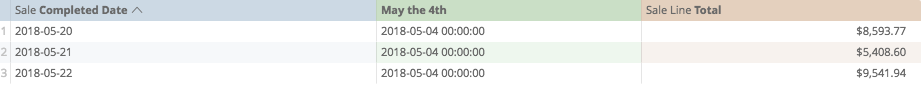# Trunc_Days (or Weeks, Months, Years)

Rounds time down to the desired closest interval.

In this instance, I have used the trunc_months function to change the sale date to the month: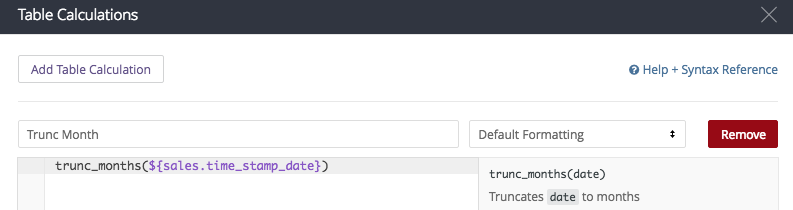My calculation looks like:
trunc_months(\${sales.time_stamp_date})

and if I've prepared it properly, my table looks like: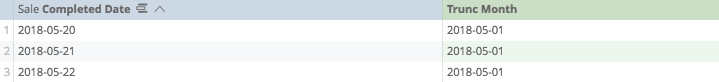Calculations Home
Post edited by josephmckeown on
Joseph McKeown
Lightspeed Analytics and reporting consultant
Lightspeed HQ